# 使用Numpy和Opencv完成图像的基本数据分析（Part II）

在上一节中，主要是介绍了图像的基本知识以及OpenCV的基本操作，具体内容参见“使用Numpy和Opencv完成基本图像的数据分析（Part I）”。这部分内容是接着上一节的内容，主要介绍一些其它的操作。

## 使用逻辑操作处理像素值

可以使用逻辑运算符创建相同大小的数组。但是，逻辑运算操作并不会创建出任何新的数组，只是将True返回给主机变量（host variable）。例如：假设在RGB图像中过滤掉一些像素值低的像素或像素值高的像素（以及其它任何条件），将RGB转换为灰度图看起来不错，但是我们目前不会对彩色图像进行这样的处理。
首先加载图像，并将其显示在屏幕上：

pic=imageio.imread('F:/demo_1.jpg')
plt.figure(figsize=(10,10))
plt.imshow(pic)
plt.show()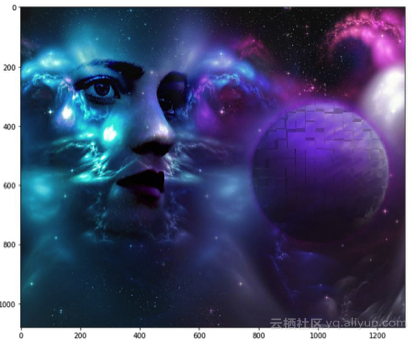注意图像的路径问题.之后需要考虑转储这个显示的图像。假设对于任何情况，我们都想要滤除掉低于某值的所有像素值，并假设该阈值设置为20。为此，我们将使用逻辑运算符来执行此任务，最终结果将返回所有索引的真值。
low_pixel=pic<20
# 检查所有值在低像素中是否正确
iflow_pixel.any()==True:
print(low_pixel.shape)

# 输出结果
(1079, 1293, 3)

正如之前所说，主机变量，一般并不使用这个名称，但在本文中引用它，这是因为它的行为只保留真值，而不是其他任何形式的值。所以，如果展示low_pixel和pic的形状，我们就会发现它们其实具有相同的形状。

print(pic.shape)
print(low_pixel.shape)

# 输出
(1079,1293,3)
(1079,1293,3)

我们使用全局比较运算符为所有像素值小于200的像素点生成低值滤波器。但是，我们也可以使用此low_pixel数组作为索引将这些低值设置为某些特定值，这些值可能高于或低于先前的像素值。

# 随机选择一个值
import random
# 加载原始图像
# 随机设置一个值，取值范围25~225
choosenpic[low_pixel]=random.randint(25,225)
# 展示图像
plt.figure(figsize=(10,10))
plt.imshow(pic)plt.show()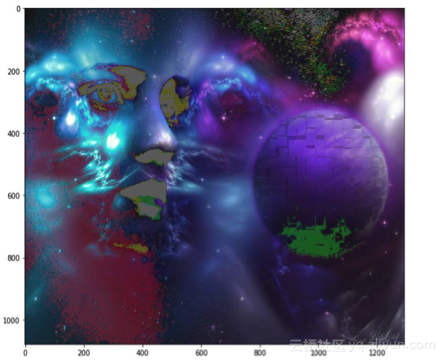## 掩膜

图像掩膜是一种图像处理技术，被广泛用于去除具有模糊边缘、透明或毛刺部分的照片背景，看起来类似于PS中的一项技术。
下面将带领读者一起创建一个圆盘形状的掩膜。首先，我们测量从图像中心到每个边界像素值的距离，在这里采用应用比较方便的半径，然后使用逻辑运算符创建一个圆盘。这个过程很简单，如下面的代码所示：

if__name__=='__main__':
# 加载图像
# 分离行和列
total_row,total_col,layers=pic.shape
''' 创建矢量，Ogrid 是一个不错的方法method of creating a

>>>ogrid[0:5,0:5]

[array([,,,,]),
array([[0, 1, 2, 3, 4]])]
'''
x,y=np.ogrid[:total_row,:total_col]
# 获取图像的中心值
cen_x,cen_y=total_row/2,total_col/2
'''

'''
distance_from_the_center=np.sqrt((x-cen_x)**2+(y-cen_y)**2)
# 选择半径值
# 使用逻辑操作符 '>'
'''

'''
pic[circular_pic]=0
plt.figure(figsize=(10,10))
plt.imshow(pic)
plt.show()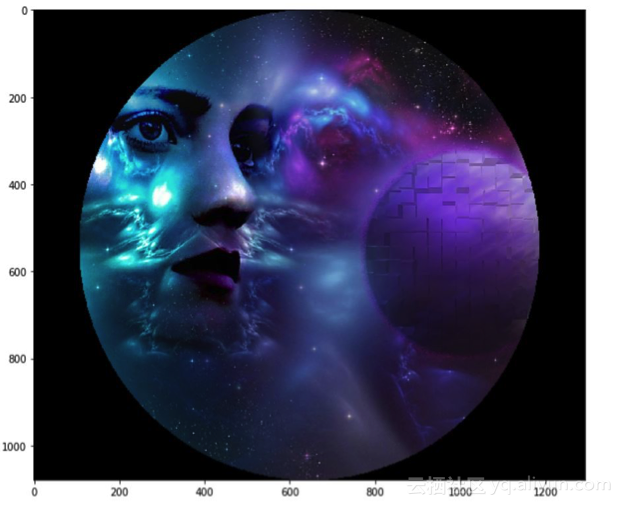## 卫星图像处理

作为edX的公开课之一，下面将介绍一些卫星图像及其处理方法，这部分内容是十分有用的，下面对其进行一些处理，做一些分析任务。

# 加载图像
plt.figure(figsize=(10,10))
plt.imshow(pic)
plt.show()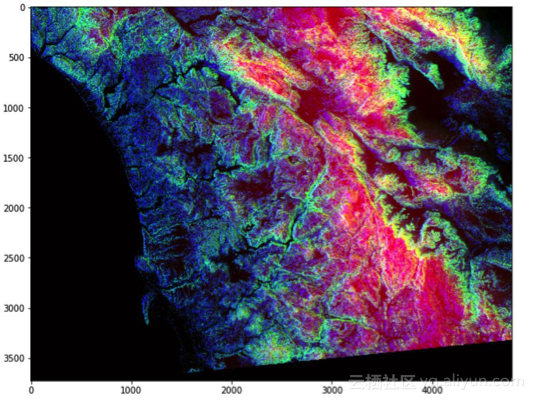下面看看它的一些基本信息：
print(f'Shape of the image {pic.shape}')

print(f'hieght {pic.shape} pixels')

print(f'width {pic.shape} pixels')

# 输出
Shapeoftheimage(3725,4797,3)

height 3725 pixels

width 4797 pixels

从中可以发现一些有趣的东西，像许多其他可视化结果一样，每个rgb层中的颜色都表示对应的内容。例如，红色强弱表示像素中地理数据点的高度，蓝色强弱表示方位的度量，而绿色表示斜率，这些颜色将有助于我们以更快、更有效的方式传达信息，而不仅是显示数字。

• 红色像素表示：高度
• 蓝色像素表示：方位
• 绿色像素表示：斜率
只需看一下这张彩色图像，训练有素的眼睛就能分辨出海拔是多少，斜率是多少，方位在哪里，所以为这些颜色加载更多含义能够表示更科学的东西，一个好的想法！

## 检测每个通道的高像素

# 只有红色通道值,像素值高于180

plt.figure(figsize=(15,15))

plt.imshow(pic)

# 只有绿色通道像素值，像素值高于180

plt.figure(figsize=(15,15))

plt.imshow(pic)

# 只有蓝色通道像素值，像素值高于180

plt.figure(figsize=(15,15))

plt.imshow(pic)

# 使用逻辑与的组合掩膜

plt.figure(figsize=(15,15))

plt.imshow(pic)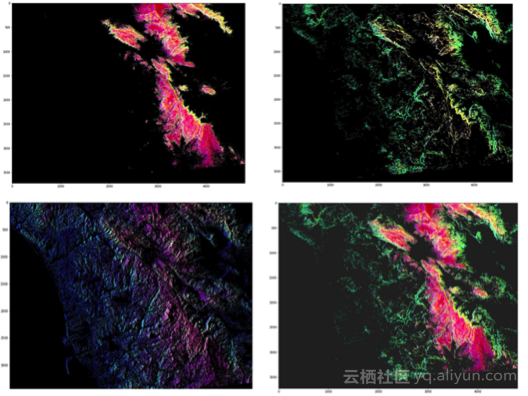## 作者信息

Mohammed Innat，机器学习和数据科学研究者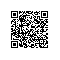使用钉钉扫一扫加入圈子
+ 订阅